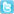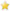Try our desktop site

Pocket PC Freeware .mobiSubscribe for RSS feedTwitter

»

KalculatorRating: 4/5
Simple scientific calculator.
Arrived: Oct 3, 2011
Found under: calculator, math, sin, cos, tan, exp, ln, pi
»

Smart AlgebraRating: 4/5
Plotting functions in polar and Cartesian coordinate systems.
Arrived: Jul 16, 2010
Found under: misc, science, math, science, plot
»

Count ItRating: 4/5
A simple application to help make forgetting your count a thing of the past.
Arrived: May 17, 2010
Found under: math, tool, memory, counting
»

SpaceTimeRating: 5/5
SpaceTime 4.0 is the most powerful mathematics program ever developed for Windows Mobile powered devices.
Arrived: Apr 26, 2010
Found under: Misc, Science, Math, Calculators, plot, graphing
»

Math Tools

Simple open-source math tools program written with Basic4PPC. Calculate the perimeter, area en volume and ABC-formula. Program is multi-language: English, Dutch and German.
Arrived: Mar 9, 2010
Found under: tools, math, calculation
»

SMath StudioRating: 5/5
Absolutely free mathematical program with &amp;amp;quot;paper&amp;amp;quot;-like interface and numerous computing features (now with integrated mathematical reference book).
Arrived: Feb 11, 2010
Found under: math, solve, science, matrix, equation, mathcad, number, Misc, Science, calculator, utilities, proffesional,
»

SMath Studio PPCRating: 5/5
Absolutely free mathematical program with "paper"-like interface and numerous computing features (now with integrated mathematical reference book).
Arrived: May 12, 2009
Found under: Misc, Science, calculator, utilities, proffesional, math
»

PocketCASRating: 5/5
PocketCAS is a free interface to the open-source Computer Algebra System giac/xcas.
Arrived: Nov 8, 2008
Found under: Productivity, Calculators, calculator, science, math, functions
»

Lemur PlotterRating: 5/5
Software for plotting 2D functions and analyzing them.
Arrived: Sep 11, 2008
Found under: Misc, Science, Math, plot, graph, analyze
»

Balance KeeperRating: 4/5
Balance Keeper is an educational tool that helps children practice addition, subtraction, logical thinking, and basic algebra.
Arrived: Jun 13, 2008
Found under: mathematics, algebra, education, utilities
Next Page »